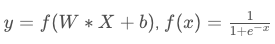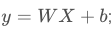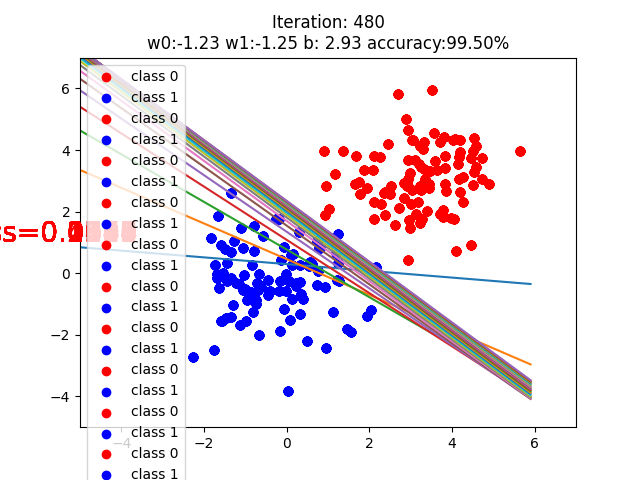深度学习模型的训练就是不断更新权值，权值的更新需要求解梯度，梯度在模型训练中是至关重要的。

• 功能：自动求取计算图所有节点变量的梯度
• tensor：用于求导的张量，如loss
• retain_graph：保存计算图；由于pytorch采用动态图机制，在每一次反向传播结束之后，计算图都会释放掉。如果想继续使用计算图，就需要设置参数retain_graph为True
• create_graph：创建导数计算图，用于高阶求导，例如二阶导数、三阶导数等等

import torch
torch.manual_seed(10)  #用于设置随机数

flag = True
# flag = False

if flag:

y = torch.mul(a, b)

y.backward()   #对y执行backward方法就可以得到x和w两个叶子节点
'''
backward()中有一个retain_graph参数，它是用来保存计算图的，如果还想执行一次反向传播 ，必须将retain_graph参数设置为True，否则代码会报错。因为如果没有设置为True，每进行一次backward之后，计算图都会被清空，没法再进行一次backward()操作

y.backward(retain_graph=True)
y.backward(retain_graph=True)
输出为：tensor([10.])
'''

import numpy as np
import tensor
flag = True
if flag:
y0 = torch.mul(a, b)  # y0 = (x+w) * (w+1)
y1 = torch.add(a, b)  # y1 = (x+w) + (w+1)
loss = torch.cat([y0, y1], dim=0)  # [y0, y1]，损失函数由两部分组成
print(w.grad)    # w = 1*5 + 2*2 = 9，输出为9

• 功能：求取梯度
• outputs：用于求导的张量，如loss；
• inputs：需要梯度的张量，如上面代码中的w；
• create_graph：创建导数计算图，用于高阶求导；
• retain_graph：保存计算图
import numpy as np
import torch
flag = True
if flag:
y = torch.pow(x, 3)  # y = x**2

flag = True
if flag:
for i in range(4):
y = torch.mul(a, b)

y.backward()

tensor([5.])
tensor([10.])
tensor([15.])
tensor([20.])

flag = True
if flag:
for i in range(4):
y = torch.mul(a, b)
y.backward()
tensor([5.])
tensor([5.])
tensor([5.])
tensor([5.])

Logistic 算法(逻辑回归算法)

逻辑回归与线性回归的区别• 逻辑回归是在线性回归的基础上加了一个激活函数sigmoid()
• 为了更好地描述分类置信度，所以采用sigmoid函数将输出映射到0-1，符合一个概率取值

机器学习的一般步骤

优化器

@本代码来源于深度之眼《pytorch框架班》
@作者：课程讲师：余老师
@如有侵权，请联系我

import torch
import torch.nn as nn
import matplotlib.pyplot as plt
import numpy as np
torch.manual_seed(10)
from math import *

# ============================ step 1/5 生成数据 ============================
sample_nums = 100
mean_value = 1.7
bias = 1.5
n_data = torch.ones(sample_nums, 2)
x0 = torch.normal(mean_value * n_data, 1) + bias      # 类别0 数据 shape=(100, 2)
y0 = torch.zeros(sample_nums)                         # 类别0 标签 shape=(100, 1)
x1 = torch.normal(-mean_value * n_data, 1) + bias     # 类别1 数据 shape=(100, 2)
y1 = torch.ones(sample_nums)                          # 类别1 标签 shape=(100, 1)
train_x = torch.cat((x0, x1), 0)
train_y = torch.cat((y0, y1), 0)

# ============================ step 2/5 选择模型 ============================
class LR(nn.Module):
def __init__(self):
super(LR, self).__init__()
self.features = nn.Linear(2, 1)
self.sigmoid = nn.Sigmoid()

def forward(self, x):
x = self.features(x)
x = self.sigmoid(x)
return x

lr_net = LR()   # 实例化逻辑回归模型

# ============================ step 3/5 选择损失函数 ============================
loss_fn = nn.BCELoss()

# ============================ step 4/5 选择优化器   ============================
lr = 0.01  # 学习率
optimizer = torch.optim.SGD(lr_net.parameters(), lr=lr, momentum=0.9)

# ============================ step 5/5 模型训练 ============================
for iteration in range(1000):

# 前向传播
y_pred = lr_net(train_x)

# 计算 loss
loss = loss_fn(y_pred.squeeze(), train_y)

# 反向传播
loss.backward()

# 更新参数
optimizer.step()

# 清空梯度

# 绘图
if iteration % 20 == 0:

correct = (mask == train_y).sum()  # 计算正确预测的样本个数
acc = correct.item() / train_y.size(0)  # 计算分类准确率

plt.scatter(x0.data.numpy()[:, 0], x0.data.numpy()[:, 1], c='r', label='class 0')
plt.scatter(x1.data.numpy()[:, 0], x1.data.numpy()[:, 1], c='b', label='class 1')

w0, w1 = lr_net.features.weight
w0, w1 = float(w0.item()), float(w1.item())
plot_b = float(lr_net.features.bias.item())
plot_x = np.arange(-6, 6, 0.1)
plot_y = (-w0 * plot_x - plot_b) / w1

plt.xlim(-5, 7)
plt.ylim(-5, 7)
plt.plot(plot_x, plot_y)

plt.text(-8, 1, 'Loss=%.4f' % loss.data.numpy(), fontdict={
'size': 20, 'color': 'red'})
plt.title("Iteration: {}\nw0:{:.2f} w1:{:.2f} b: {:.2f} accuracy:{:.2%}".format(iteration, w0, w1, plot_b, acc))
plt.legend()
#plt.show()
plt.pause(0.5)
if acc > 0.99:
plt.show()
breakfileinput 时间_在使用vue时候使用bootstrap-fileinput_weixin_39760721的博客-程序员宅基地

bootstrap-fileinput这个控件不错，首先感谢这个控件的作者在使用vue的时候当然是不太愿意再回到原始的dom操作时代\$(...)之类写一大堆整个项目中完全重复的默认配置。我希望简单，像这样：实际上的确简单了不少，不过缺点是同事在接手这个逻辑的时候需要一定的熟悉时间，当然前端大佬不需要。这也是在我探索vue的过程中找到的一般性用法，前端大佬可在评论区吐槽，期待有更优的方式，拜读，学习...

linux默认进去什么目录,linux系统默认目录_xander Sun的博客-程序员宅基地

linux系统默认目录/bin：此目录放置操作系统所需使用的各种命令程序。例如cp、ls、tar、ps等常用命令，还有各种不同的，如bash、tcsh等。/boot：系统启动时必须读取的文件，包括系统核心文件。/dev：保存着外围设备代号的文件。例如硬盘/dev/hda2等，这些文件比较特殊，实际它们都指向所代表的外围设备。/etc：保存与系统设置、管理相关的文件。例如记录用户帐号名称的passw...

linux ping大包指令,linux下ping命令使用详解，_无知天的博客-程序员宅基地

linux下ping命令使用详解，•ping命令一般用于检测网络通与不通，也叫时延，其值越大，速度越慢PING(PacketInternetGrope)，因特网包探索器，用于测试网络连接量的程序。•ping发送一个ICMP回声请求消息给目的地并报告是否收到所希望的ICMP回声应答。它是用来检查网络是否通畅或者网络连接速度的命令。•原理：网络上的机器都有唯一确定的IP地址，我们给目标IP地址发送一个...

hp服务器修改阵列,HP服务器阵列配置教程(适合初学者)_黑忠的博客-程序员宅基地

HP服务器阵列配置教程(适合初学者) (3页) 本资源提供全文预览，点击全文预览即可全文预览,如果喜欢文档就下载吧，查找使用更方便哦！9.90 积分注：此文档完全由编者的经验而成，仅供大家参考，故有不正确之处请大家见谅，编者也不承担任何责任。注：此文档完全由编者的经验而成，仅供大家参考，故有不正确之处请大家见谅，编者也不承担任何责任。 ProLiant 系列服务器系列服务器 F8 阵列配置过程阵...

es获得所有index和type_Libbytian-程序员宅基地_es查看所有index

1.获得所有indexrest方式：curl 'localhost:9200/_cat/indices?v'JAVA方式：GetIndexResponse response = client.admin().indices().prepareGetIndex().execute().actionGet(); System.out.println(response.getIndices()...

JScript中的undefined和"undefined"_weixin_34384557的博客-程序员宅基地

JScript中用于表示未定义的undefined标识符到底是表示什么未定义？它和"undefined"(包括"在内)又有什么区别和联系呢？为什么有的时候可以使用undefined来和变量做比较，而有的时候又不行呢？ undefined和"undefined"的区别大家一眼就能看出来。在一般的认知下，我们认为undefined是JScript...